Thank you for visiting nature.com. You are using a browser version with limited support for CSS. To obtain the best experience, we recommend you use a more up to date browser (or turn off compatibility mode in Internet Explorer). In the meantime, to ensure continued support, we are displaying the site without styles and JavaScript.

# Cooper-pair-mediated coherence between two normal metals

## Abstract

Two electrons bound in a singlet state have long provided a conceptual and pedagogical framework for understanding the non-local nature of entangled quantum objects. As bound singlet electrons separated by a coherence length of up to several hundred nanometres occur naturally in conventional Bardeen–Cooper–Schrieffer superconductors in the form of Cooper pairs, recent theoretical investigations1,2,3,4,5,6,7,8,9 have focused on whether electrons in spatially separated normal-metal probes placed within a coherence length of each other on a superconductor can be quantum mechanically coupled by the singlet pairs. This coupling is predicted to occur through the non-local processes of elastic cotunnelling and crossed Andreev reflection. In crossed Andreev reflection, the constituent electrons of a Cooper pair are sent into different normal probes while retaining their mutual coherence. In elastic cotunnelling, a sub-gap electron approaching the superconductor from one normal probe undergoes coherent, long-range tunnelling to the second probe that is mediated by the Cooper pairs in the condensate. Here, we present experimental evidence for coherent, non-local coupling between electrons in two normal metals linked by a superconductor. The coupling is observed in non-local resistance oscillations that are periodic in an externally applied magnetic flux.

## Main

Three key predicted signatures of the elastic cotunnelling and crossed Andreev reflection (CAR) processes, shown schematically in Fig. 1, are (1) the coupling created between the normal probes is non-local—no current need be sent between them, (2) the resultant non-local signals decay rapidly as the probe separation is increased, exponentially over a superconducting coherence length ξS or faster4,5,7 and (3) the processes create quantum phase coherence between the two probes. Previous experiments looking for evidence of elastic cotunnelling and CAR have used normal–superconductor–normal and ferromagnet–superconductor–ferromagnet devices. A current is sent across one normal–superconductor or ferromagnet–superconductor interface and non-local voltages are measured on the other normal or ferromagnet probe located less than a coherence length from the interface10,11,12. Although the measured non-local signals exhibit behaviour consistent with predictions (1) and (2), the coherent nature of the non-local signals has not been demonstrated. To establish the presence of non-local coherence, we have attached a hybrid normal–superconducting loop known as an Andreev interferometer13,14,15 to one of the normal probes in a normal–superconductor–normal device. Modifying the phase of the electrons in the normal part of the Andreev interferometer by threading a magnetic flux through the loop leads to oscillations in the resistance of the loop with a period equal to the superconducting flux quantum Φ0=h/2e. Periodic oscillations can also be seen in the non-local resistance measured using normal probes placed off the interferometer, but coupled to it by a superconducting wire of length comparable to ξS. These oscillations are the result of CAR/elastic-cotunnelling processes that couple the non-local probes to flux-dependent quasiparticle currents in the normal arm of the Andreev interferometer.

Figure 2a shows a scanning electron micrograph of a hybrid loop device. Although three devices with slightly different geometries were measured (see Supplementary Fig. S1), all show consistent results and here we concentrate on a single device with the geometry shown in Fig. 2a. At the centre of the device is a square loop 1.7 μm on each side formed from lines of 80 nm width. The loop is composed of 80-nm-thick Al lines on three sides and a 50-nm-thick Au wire on one side. Two superconducting leads attached to the loop on the left and two normal leads on the right serve as current and voltage probes for four-terminal measurements of the loop. Extra normal-metal voltage probes are placed on the right side of the loop at the top and bottom corners, and non-locally, just off the loop. The non-local probes are coupled to the normal part of the loop through a superconducting section of length 110 nm, comparable to the superconducting coherence length of Al in the dirty limit16.

Figure 2b and the inset of Fig. 2a show data for the resistance of the loop using the current and voltage lead configuration marked in Fig. 2a. This is the local measurement configuration. As a function of temperature, the resistance undergoes a sharp drop at 1.2 K as the Al sections become superconducting, and the remaining resistance of the normal side gradually decreases as the loop is cooled further owing to the superconducting proximity effect. At 70 mK, the resistance begins to decline more sharply, although even at 14 mK, the base temperature of our refrigerator, the loop has a residual resistance above 1 Ω. The energy scale at which the superconducting proximity effect encompasses the normal section of the loop is given by the Thouless energy Ec=D/L2, where L is the length of the normal section and D is the electron diffusion coefficient. Using measurements of a simultaneously fabricated Au wire to determine D=110 cm2 s−1 gives Ec=2.5 μeV for the L=1.7 μm normal side of the loop. This energy corresponds to a temperature of 30 mK, indicating that the low-temperature resistance drop of the loop is due to its transitioning into the correlation regime, where the time it takes electrons to traverse the normal-metal section is less than the decoherence time due to thermal energy. This regime can also be seen in the differential resistance measurements of the loop at 14 mK, where a sharp increase can be seen over a ±1 μA range. Robust coherent behaviour in the hybrid loop such as the large h/2e periodic resistance oscillations shown as an inset to Fig. 2a is observed in this correlation regime only below ±1 μA d.c. current and 70 mK.

As shown in Fig. 3a, to measure a phase-coherent non-local signal due to elastic cotunnelling and CAR, a phase-dependent quasiparticle current Iqp(Φ) needs to be injected from one normal probe into the superconductor while a non-local voltage is measured on a second probe VN relative to the superconductor potential VS. If an observed non-local voltage is due to a phase-coherent process, tuning the phase of Iqp(Φ) will reveal a phase-dependent non-local voltage. Iqp(Φ) is established by embedding the first probe in our interferometer (Fig. 3b) and adding a small d.c. current below 1 μA that is modulated by an a.c. measurement current. As discussed below, the small but finite d.c. current creates a non-equilibrium quasiparticle distribution in the normal arm of the loop that is necessary in these devices to establish Iqp(Φ) and the resulting phase-dependent non-local voltages. Similar to the local measurement above, the phase of Iqp(Φ) is tuned by an external flux through the interferometer loop. Although the current path of the local configuration marked in Fig. 2a may be used, any path along or intersecting the normal arm of the interferometer produces a non-equilibrium quasiparticle distribution in the arm, leading to similar qualitative effects. These effects are also independent of any non-equilibrium distribution induced in the non-local voltage probes (see Supplementary Fig. S2). We present results for the current path of Fig. 3b, where the current crosses only the centre of the loop, as it helps to emphasize the non-local nature of the measurement and illuminates the physics of the device.

Using this current configuration, we measure the differential voltage signals (normalized by the a.c. measurement current amplitude) on the non-local probes off the loop (subscript N) as well as the leads on the corner of the loop (subscript C) relative to the superconductor potential VS. We also compare the signals seen at the top and bottom of the loop (superscripts T and B). Figure 3 exhibits the flux-dependent voltages on these four probes. The signals on the non-local probes, VNT and VNB, show oscillations with the Φ0 flux period dictated by the geometry of the loop. Thus, the quasiparticles in the non-local probes off the loop are being coupled to the phase-coherent processes on the loop, consistent with the predicted elastic cotunnelling and CAR effects. In addition to the coherent nature of these non-local signals, the decay of this signal along the superconductor confirms that they are due to elastic cotunnelling and CAR. The oscillations on the VCT and VCB corner leads are identical in behaviour to the oscillations on their respective non-local leads, but with an amplitude six times as large. The strong attenuation of the signals along the 110 nm from the corner to non-local leads is consistent with an expected dependence on the superconducting coherence length ξS for elastic cotunnelling and CAR processes4,5,7.

As we noted above, the non-local signals shown in Fig. 3 arise from a phase-dependent quasiparticle current Iqp(Φ) in the normal arm of the loop. The origin of Iqp(Φ) can be understood by examining in detail the effect of the non-equilibrium quasiparticle distribution induced by the d.c. current. Within the framework of the quasiclassical theory of superconductivity (see the Methods section), the current between the two normal–superconductor interfaces can be expressed as17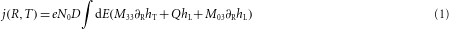Here, N0 is the quasiparticle density of states at the Fermi energy, T is the temperature, M33 and M03 are normalized diffusion coefficients that depend on the energy E and spatial coordinates R and Q is the energy-dependent spectral supercurrent. hT and hL are space-dependent quasiparticle distribution functions that have the equilibrium values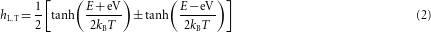at a reservoir (either superconducting or normal) with an applied voltage V. In particular, at the superconducting reservoirs at the normal–superconductor interface, where we assume V is 0 with no loss of generality, hT=0 and hL=tanh(E/2kBT).

At low temperatures, where the quasiparticle inelastic scattering length is very long, application of a d.c. current as shown in Fig. 3b will result in non-equilibrium space-dependent distribution functions hT and hL in the normal arm of the interferometer. As has already been demonstrated experimentally in superconductor–normal–superconductor junctions, this can affect the total supercurrent through the second term in the brackets in equation (1), even leading to reversal in sign of the critical current, the so-called tunable π-junction18,19,20. In our sample, the application of the d.c. current results in the value of hL at the midpoint of the normal arm of the Andreev interferometer being different from its value at either normal–superconductor interface. As the spectral supercurrent Q is conserved, the total supercurrent, given by the integral of Q hL over the energy E also varies as a function of position along the normal arm. The conservation of the total current then implies that there is a compensating quasiparticle current arising from the first and third terms in the brackets in equation (1) (ref. 21).

Simulations based on the quasiclassical theory (see the Methods section) show that hL varies appreciably only in the vicinity of the normal–superconductor interfaces, so that these quasiparticle currents are also appreciable only in the vicinity of the normal–superconductor interfaces. As the supercurrent is periodic in the applied flux, the induced Iqp is also periodic in the applied flux, giving rise to a periodic non-local resistance through CAR/elastic-cotunnelling processes7. Figure 4b shows Iqp arising from the first term in the brackets in equation (1) near the normal–superconductor interface as a function of the phase difference Δφ between the two normal–superconductor interfaces at a finite voltage V =3Ec applied between the normal reservoirs. The calculated Iqp is periodic and antisymmetric in Δφ. Whereas the calculated circulating currents are approximately sinusoidal in Δφ, the measured non-local voltages have a slightly more sawtooth dependence on the applied flux; this is due to self-flux effects associated with the finite inductance of the Andreev interferometer22,23,24. Furthermore, the fact that the signals measured at the top and bottom corners are of opposite sign is consistent with the fact that Iqp goes from the superconductor to the normal arm of the Andreev interferometer at one normal–superconductor interface, whereas it goes from the normal arm into the superconductor at the second normal–superconductor interface.

Figure 4c shows VCT as a function of the injected d.c. current with an applied flux of Φ0/4 through the interferometer, approximately the flux at which the maximum signal is observed. The signal oscillates as a function of the d.c. current, vanishing at zero d.c. current and high d.c. currents. This dependence on the d.c. current is strongly reminiscent of the behaviour of the supercurrent in multi-terminal normal–superconductor devices that has been predicted and observed experimentally18,19,20. Figure 4d shows the calculated differential resistance as a function of the voltage V (proportional to the applied d.c. current), which reproduces qualitatively the behaviour we see in our experiments. Our experiments show, and the simulations predict, that the quasiparticle current Iqp is present only when there is a non-equilibrium distribution in the normal arm of the loop. It is only under non-equilibrium conditions that a conversion of supercurrent to quasiparticle current can occur.

## Methods

### Experimental.

Our devices are fabricated using photo and electron-beam lithography on Si substrates with 300 nm SiO2 insulating layers. The critical features are patterned in spun methyl methacrylate/polymethyl methacrylate bilayers using a Tescan MIRA electron microscope. The 99.999% pure Au and Al films are deposited in an Edwards thermal evaporator with a base pressure of 3×10−7 torr. Before the Au deposition, in situ O2+ plasma etching is carried out to clean the substrate, and before the Al deposition in situ Ar+ plasma etching is carried out to ensure clean interfaces between the two materials. After the Al deposition, the devices are loaded into an Oxford dilution refrigerator and cooled to 77 K within a few hours to prevent degradation of the interfaces. Measurements of our interfaces show a barrier resistivity of 1.9 μm2 Ω, comparable to the Sharvin resistivity.

Measurements were carried out using PAR 124 lock-in amplifiers with modified Adler–Jackson resistance bridges25. The bridges can introduce small, 100 mΩ, offsets to the data, which are removed by cross-checking the measured resistance using a true four-terminal measurement with a current source and lock-in amplifier. Low-frequency (<100 Hz) a.c. measurement currents at two different frequencies and amplitudes of 20 and 100 nA were used for local and non-local measurements respectively, with 5 nA currents used to check against the possibility of effects due to heating. To remove extraneous noise signals, first-stage amplification of the measurement signals occurred in a mu-metal-shielded enclosure attached to the cryostat.

### Theoretical

The simulations were done by solving the Usadel equations of quasiclassical superconductivity in the so-called θ parametrization for the geometry shown in the inset to Fig. 4b. Using the formalism of ref. 17, these equations can be written in the normal wires as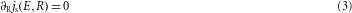and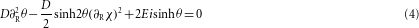Here, χ is the complex gauge-invariant phase, and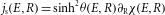where the spectral supercurrent Q is related to js by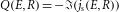. In the normal wire, RQ=0. In addition, we have the condition that the spectral electronic current j(E,R)=M33RhT+Q hL+M03RhL and the spectral thermal current jth(E,R)=M00RhL+Q hT+M30RhT are conserved, that is, Rj(E,R)=0 and Rjth(E,R)=0. Here, the normalized diffusion coefficients M are given by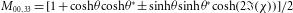,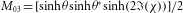and M30=−M03.

The solutions to these coupled equations are obtained by first solving equations (3) and (4) for the complex θ and χ for the geometry of the inset to Fig. 4b along all four normal wires of the cross, using a numerical relaxation technique and appropriate boundary conditions. The boundary conditions at a normal reservoir are that θ and χ vanish. The phase difference Δφ is dropped symmetrically between the superconducting reservoirs, being set to Δφ/2 at one superconducting reservoir, and −Δφ/2 at the other, as shown in the inset to Fig. 4b. The boundary condition for θ at a superconducting reservoir is given by θS0=−i(π/2)+(1/2)ln[(|Δ|+E)/(|Δ|−E)], if E<|Δ|, and θS0=(1/2)ln[(E+|Δ|)/(E−|Δ|)], if E>|Δ|, where Δ is the gap in the superconducting reservoir. The boundary conditions at the node where all four wires meet are that θ and χ are continuous, and that the sum of their derivatives is equal to 0.

After θ and χ are obtained, the kinetic equations Rj(E,R)=0 and Rjth(E,R)=0 are then solved using a numerical relaxation technique, subject to the boundary conditions on hT and hL at the reservoirs given by equation (2). We assume the voltage applied to the normal reservoirs is dropped symmetrically between them, as shown in the inset to Fig. 4b. The quasiparticle current shown in Fig. 4b is given by the integral of M33RhT over the energy E, that is, the first term in the brackets in equation (1); the supercurrent is given by the second term. (The contribution of the third term is much smaller than that of the first term, but has a similar qualitative dependence.) To calculate the differential resistance dV/dI shown in Fig. 4d, we take the derivative of Iqp calculated as above with respect to V. As the voltage V is linearly related to the d.c. current by the resistance of the normal wires connected to the normal reservoirs, and the non-local voltage measured should also be linearly related to the quasiparticle current in the non-local normal arm through elastic-cotunnelling/CAR, this quantity is the same as dV/dI to within a numerical factor.

## References

1. Byers, J. M. & Flatté, M. E. Probing spatial correlations with nanoscale two-contact tunneling. Phys. Rev. Lett. 74, 306–309 (1995).

2. Deutscher, G. & Feinberg, D. Coupling superconducting-ferromagnetic point contacts by Andreev reflections. Appl. Phys. Lett. 76, 487–489 (2000).

3. Falci, G., Feinberg, D. & Hekking, F. W. J. Correlated tunneling into a superconductor in a multiprobe hybrid structure. Europhys. Lett. 54, 255–261 (2001).

4. Feinberg, D. Andreev scattering and cotunneling between two superconductor-normal metal interfaces: The dirty limit. Euro. Phys. J. B 36, 419–422 (2003).

5. Chtchelkatchev, N. M. Superconducting spin filter. JETP Lett. 78, 230–235 (2003).

6. Mélin, R. & Feinberg, D. Sign of the crossed conductances at a ferromagnet/superconductor/ferromagnet double interface. Phys. Rev. B 70, 174509 (2004).

7. Brinkman, A. & Golubov, A. A. Crossed Andreev reflection in diffusive contacts: Quasiclassical Keldysh-Usadel formalism. Phys. Rev. B 74, 214512 (2006).

8. Morten, J. P., Brataas, A. & Belzig, W. Circuit theory for crossed Andreev reflection and nonlocal conductance. Appl. Phys. A 89, 609–612 (2007).

9. Levy Yeyati, A., Bergeret, F. S., Martín-Rodero, A. & Klapwijk, T. M. Entangled Andreev pairs and collective excitations in nanoscale superconductors. Nature Phys. 3, 455–459 (2007).

10. Beckmann, D., Weber, H. B. & Löhneysen, H. v. Evidence for crossed Andreev reflection in superconductor-ferromagnet hybrid structures. Phys. Rev. Lett. 93, 197003 (2004).

11. Russo, S., Kroug, M., Klapwijk, T. M. & Morpurgo, A. F. Experimental observation of bias-dependent nonlocal Andreev reflection. Phys. Rev. Lett. 95, 027002 (2005).

12. Cadden-Zimansky, P. & Chandrasekhar, V. Nonlocal correlations in normal-metal superconducting systems. Phys. Rev. Lett. 97, 237003 (2006).

13. Nakano, H. & Takayanagi, H. Quasiparticle interferometer controlled by quantum-correlated Andreev reflection. Phys. Rev. B 47, 7986–7994 (1993).

14. de Vegvar, P. G. N., Fulton, T. A., Mallison, W. H. & Miller, R. E. Mesoscopic transport in tunable Andreev interferometers. Phys. Rev. Lett. 73, 1416–1419 (1994).

15. Pothier, H., Gueron, S., Esteve, D. & Devoret, M. H. Flux-modulated Andreev current caused by electronic interference. Phys. Rev. Lett. 73, 2488–2491 (1994).

16. Fujiki, H. et al. Nonlinear resistivity in the mixed state of superconducting aluminum films. Physica C 297, 309–316 (1998).

17. Chandrasekhar, V. in Superconductivity: Vol. 1: Conventional and High Temperature Superconductors (eds Bennemann, K. H. & Ketterson, J. B.) 279–313 (Springer, 2008).

18. Baselmans, J. J. A., Morpurgo, A. F., van Wees, B. J. & Klapwijk, T.M. Reversing the direction of the supercurrent in a controllable Josephson junction. Nature 397, 43–45 (1999).

19. Shaikhaidarov, R., Volkov, A. F., Takayanagi, H., Petrashov, V. T. & Delsing, P. Josephson effects in a superconductor normal-metal mesoscopic structure with a dangling superconducting arm. Phys. Rev. B 62, R14 649–652 (2000).

20. Huang, J., Pierre, F., Heikkilä, T. T., Wilhelm, F. K. & Birge, N. O. Observation of a controllable π junction in a 3-terminal Josephson device. Phys. Rev. B 66, 020507(R) (2002).

21. Kogan, V.R., Pavlovskii, V.V. & Volkov, A.F. Electron–hole imbalances in superconductor/normal-metal mesoscopic structures. Europhys. Lett. 59, 875–881 (2002).

22. Büttiker, M. & Klapwijk, T. M. Flux sensitivity of a piecewise normal and superconducting metal loop. Phys. Rev. B 33, 5114–5117 (1986).

23. Cayssol, J., Kontos, T. & Montambaux, G. Isolated hybrid normal/superconducting ring in a magnetic flux: From persistent current to Josephson current. Phys. Rev. B 67, 184508 (2003).

24. Zou, J. et al. Influence of supercurrents on low-temperature thermopower in mesoscopic N/S structures. J. Low Temp. Phys. 146, 193–212 (2007).

25. Adler, J. G. & Jackson, J. E. System for observing small nonlinearities in tunnel junctions. Rev. Sci. Inst. 37, 1049–1054 (1966).

## Acknowledgements

This research was conducted with support from the National Science Foundation under grant No. DMR-0604601. We thank A. A. Golubov, A. D. Zaikin and M. R. Norman for their discussions.

## Author information

Authors

### Corresponding author

Correspondence to V. Chandrasekhar.

## Supplementary information

### Supplementary Information

Supplementary Information (PDF 565 kb)

## Rights and permissions

Reprints and Permissions

Cadden-Zimansky, P., Wei, J. & Chandrasekhar, V. Cooper-pair-mediated coherence between two normal metals. Nature Phys 5, 393–397 (2009). https://doi.org/10.1038/nphys1252

• Accepted:

• Published:

• Issue Date:

• DOI: https://doi.org/10.1038/nphys1252

• ### Real-time observation of Cooper pair splitting showing strong non-local correlations

• Antti Ranni
• Fredrik Brange
• Ville F. Maisi

Nature Communications (2021)

• ### High Efficiency CVD Graphene-lead (Pb) Cooper Pair Splitter

• I. V. Borzenets
• Y. Shimazaki
• S. Tarucha

Scientific Reports (2016)

• ### Coherent conductors at a distance

• Matthias Eschrig

Nature Physics (2009)

## Search